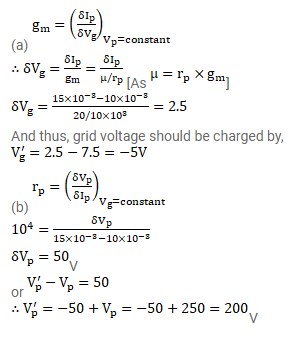# The plate resistance and the amplification factor of

Question:

The plate resistance and the amplification factor of a triode are $10 \mathrm{k} \Omega$ and 20 . The tube is operated at plate voltage $250 \mathrm{~V}$ and grid voltage $-7.5 \mathrm{~V}$. The plate current is $10 \mathrm{~mA}$.

(a) To what value should the grid voltage be changed so as to increase the plate current to $15 \mathrm{~mA}$ ?

(b) To what value should the plate voltage be changed to take the plate current back to $10 \mathrm{~mA}$ ?

Solution: